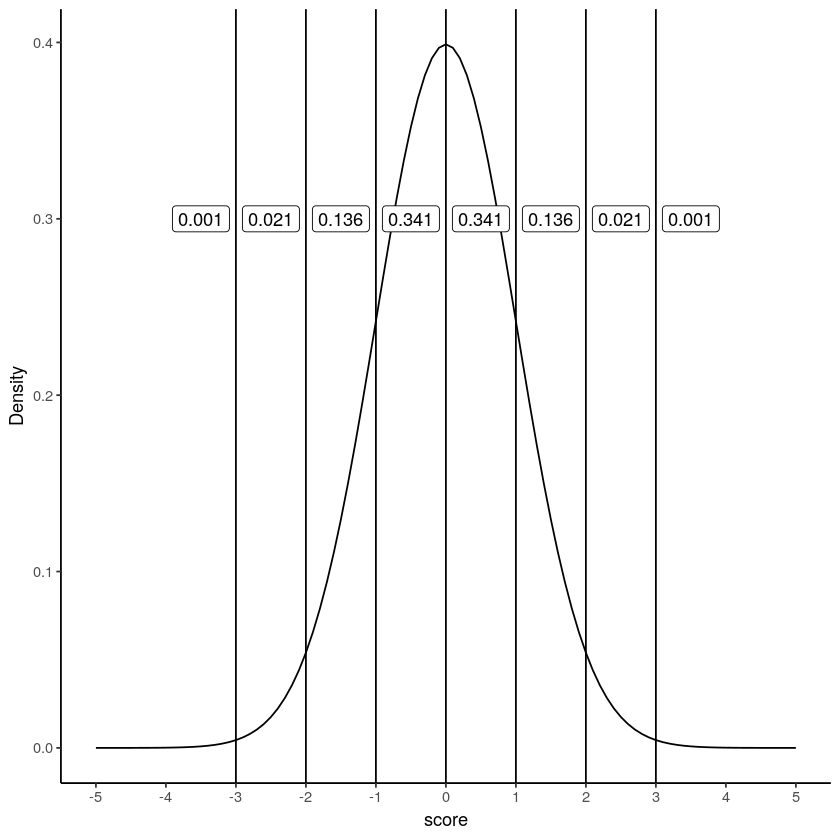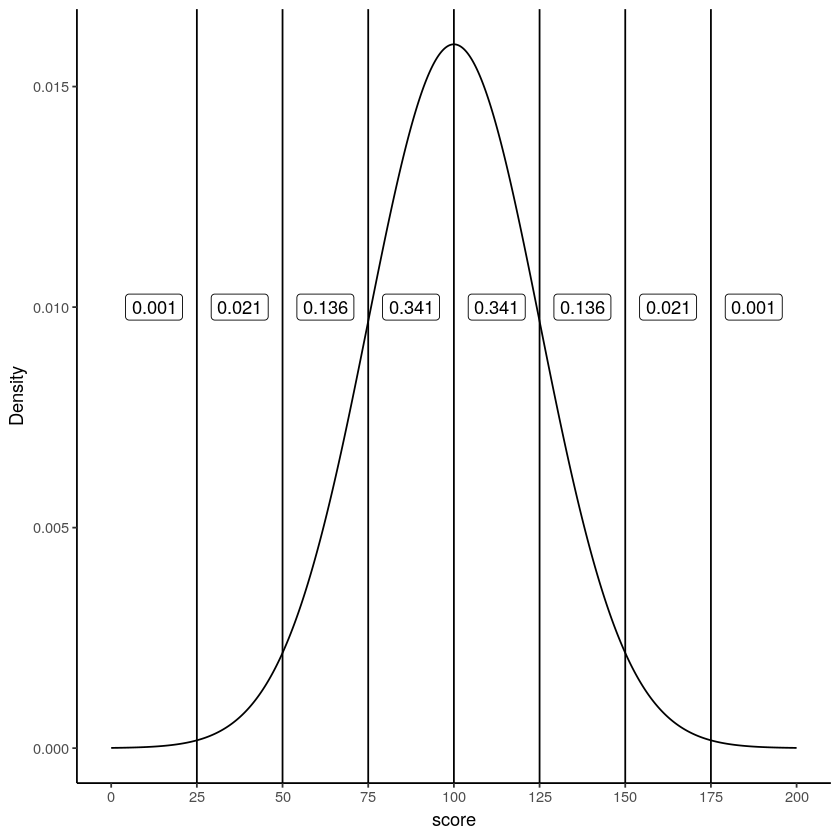# 4.12: z-scores

$$\newcommand{\vecs}{\overset { \rightharpoonup} {\mathbf{#1}} }$$ $$\newcommand{\vecd}{\overset{-\!-\!\rightharpoonup}{\vphantom{a}\smash {#1}}}$$$$\newcommand{\id}{\mathrm{id}}$$ $$\newcommand{\Span}{\mathrm{span}}$$ $$\newcommand{\kernel}{\mathrm{null}\,}$$ $$\newcommand{\range}{\mathrm{range}\,}$$ $$\newcommand{\RealPart}{\mathrm{Re}}$$ $$\newcommand{\ImaginaryPart}{\mathrm{Im}}$$ $$\newcommand{\Argument}{\mathrm{Arg}}$$ $$\newcommand{\norm}{\| #1 \|}$$ $$\newcommand{\inner}{\langle #1, #2 \rangle}$$ $$\newcommand{\Span}{\mathrm{span}}$$ $$\newcommand{\id}{\mathrm{id}}$$ $$\newcommand{\Span}{\mathrm{span}}$$ $$\newcommand{\kernel}{\mathrm{null}\,}$$ $$\newcommand{\range}{\mathrm{range}\,}$$ $$\newcommand{\RealPart}{\mathrm{Re}}$$ $$\newcommand{\ImaginaryPart}{\mathrm{Im}}$$ $$\newcommand{\Argument}{\mathrm{Arg}}$$ $$\newcommand{\norm}{\| #1 \|}$$ $$\newcommand{\inner}{\langle #1, #2 \rangle}$$ $$\newcommand{\Span}{\mathrm{span}}$$$$\newcommand{\AA}{\unicode[.8,0]{x212B}}$$

We are now in a position to combine some of things we’ve been talking about in this chapter, and introduce you to a new tool, z-scores. It turns out we won’t use z-scores very much in this textbook. However, you can’t take a class on statistics and not learn about z-scores.

The first thing we show you seems to be something that many students remember from their statistics class. This thing is probably remembered because instructors may test this knowledge many times, so students have to learn it for the test. Let’s look at this thing. We are going to look at a normal distribution, and we are going to draw lines through the distribution at 0, +/- 1, +/-2, and +/- 3 standard deviations from the mean:Figure $$\PageIndex{1}$$: A normal distribution. Each line represents a standard deviation from the mean. The labels show the proportions of scores that fall between each bar.

The figure shows a normal distribution with mean = 0, and standard deviation = 1. We’ve drawn lines at each of the standard deviations: -3, -2, -1, 0, 1, 2, and 3. We also show some numbers in the labels, in between each line. These numbers are proportions. For example, we see the proportion is .341 for scores that fall between the range 0 and 1. Scores between 0 and 1 occur 34.1% of the time. Scores in between -1 and 1, occur 68.2% of the time, that’s more than half of the scores. Scores between 1 and occur about 13.6% of the time, and scores between 2 and 3 occur even less, only 2.1% of the time.

Normal distributions always have these properties, even when they have different means and standard deviations. For example, take a look at this normal distribution, it has a mean =100, and standard deviation =25.Figure $$\PageIndex{2}$$: A normal distribution. Each line represents a standard deviation from the mean. The labels show the proportions of scores that fall between each bar.

Now we are looking at a normal distribution with mean = 100 and standard deviation = 25. Notice that the region between 100 and 125 contains 34.1% of the scores. This region is 1 standard deviation away from the mean (the standard deviation is 25, the mean is 100, so 25 is one whole standard deviation away from 100). As you can see, the very same proportions occur between each of the standard deviations, as they did when our standard deviation was set to 1 (with a mean of 0).

## Idea behind z-scores

Sometimes it can be convenient to transform your original scores into different scores that are easier to work with. For example, if you have a bunch of proportions, like .3, .5, .6, .7, you might want to turn them into percentages like 30%, 50%, 60%, and 70%. To do that you multiply the proportions by a constant of 100. If you want to turn percentages back into proportions, you divide by a constant of 100. This kind of transformation just changes the scale of the numbers from between 0-1, and between 0-100. Otherwise, the pattern in the numbers stays the same.

The idea behind z-scores is a similar kind of transformation. The idea is to express each raw score in terms of it’s standard deviation. For example, if I told you I got a 75% on test, you wouldn’t know how well I did compared to the rest of the class. But, if I told you that I scored 2 standard deviations above the mean, you’d know I did quite well compared to the rest of the class, because you know that most scores (if they are distributed normally) fall below 2 standard deviations of the mean.

We also know, now thanks to the central limit theorem, that many of our measures, such as sample means, will be distributed normally. So, it can often be desirable to express the raw scores in terms of their standard deviations.

Let’s see how this looks in a table without showing you any formulas. We will look at some scores that come from a normal distirbution with mean =100, and standard deviation = 25. We will list some raw scores, along with the z-scores

raw z
25 -3
50 -2
75 -1
100 0
125 1
150 2
175 3

Remember, the mean is 100, and the standard deviation is 25. How many standard deviations away from the mean is a score of 100? The answer is 0, it’s right on the mean. You can see the z-score for 100, is 0. How many standard deviations is 125 away from the mean? Well the standard deviation is 25, 125 is one whole 25 away from 100, that’s a total of 1 standard deviation, so the z-score for 125 is 1. The z-score for 150 is 2, because 150 is two 25s away from 100. The z-score for 50 is -2, because 50 is two 25s away from 100 in the opposite direction. All we are doing here is re-expressing the raw scores in terms of how many standard deviations they are from the mean. Remember, the mean is always right on target, so the center of the z-score distribution is always 0.

## Calculating z-scores

To calculate z-scores all you have to do is figure out how many standard deviations from the mean each number is. Let’s say the mean is 100, and the standard deviation is 25. You have a score of 97. How many standard deviations from the mean is 97?

First compute the difference between the score and the mean:

$97-100 = -3 \nonumber$

Alright, we have a total difference of -3. How many standard deviations does -3 represent if 1 standard deviation is 25? Clearly -3 is much smaller than 25, so it’s going to be much less than 1. To figure it out, just divide -3 by the standard deviation.

$\frac{-3}{25} = -.12 \nonumber$

Our z-score for 97 is -.12.

Here’s the general formula:

$z = \frac{\text{raw score} - \text{mean}}{\text{standard deviation}} \nonumber$

So, for example if we had these 10 scores from a normal distribution with mean = 100, and standard deviation =25

72.23  73.48  96.25  91.60  56.84 105.56 128.96  91.33  70.96 120.23


The z-scores would be:

-1.1108 -1.0608 -0.1500 -0.3360 -1.7264  0.2224  1.1584 -0.3468
-1.1616  0.8092


Once you have the z-scores, you could use them as another way to describe your data. For example, now just by looking at a score you know if it is likely or unlikely to occur, because you know how the area under the normal curve works. z-scores between -1 and 1 happen pretty often, scores greater than 1 or -1 still happen fairly often, but not as often. And, scores bigger than 2 or -2 don’t happen very often. This is a convenient thing to do if you want to look at your numbers and get a general sense of how often they happen.

Usually you do not know the mean or the standard deviation of the population that you are drawing your sample scores from. So, you could use the mean and standard deviation of your sample as an estimate, and then use those to calculate z-scores.

Finally, z-scores are also called standardized scores, because each raw score is described in terms of it’s standard deviation. This may well be the last time we talk about z-scores in this book. You might wonder why we even bothered telling you about them. First, it’s worth knowing they are a thing. Second, they become important as your statistical prowess becomes more advanced. Third, some statistical concepts, like correlation, can be re-written in terms of z-scores, and this illuminates aspects of those statistics. Finally, they are super useful when you are dealing with a normal distribution that has a known mean and standard deviation.

This page titled 4.12: z-scores is shared under a CC BY-SA 4.0 license and was authored, remixed, and/or curated by Matthew J. C. Crump via source content that was edited to the style and standards of the LibreTexts platform; a detailed edit history is available upon request.